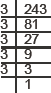#### EXERCISE

##### Type - 1
###### Question 1 :
Find the smallest number by which each of the following numbers must be multiplied to obtain a perfect cube.
(i) 243
(ii) 256
(iii) 72
(iv) 675
###### Formulae used :

Distance Formula to find distance between two points (x1,y1) and (x2,y2) is :                     D = √[(x2 – x1)2 + (y2 – y1)2 ]

###### Solution :
(i) We have 243 = 3 × 3 × 3 × 3 × 3The prime factor 3 is not a group of three.
∴ 243 is not a perfect cube.
Now,  × 3 = [3 × 3 × 3 × 3 × 3] × 3
or 729 =3 × 3 × 3 × 3 × 3 × 3
Now, 729 becomes a perfect cube.
Thus, the smallest required number to multiply 243 to make it a perfect cube is 3.
##### Class Work
Q.1.   Find the distance between the  following pairs of points:
(i)   (2, 3), (4, 1)
(ii)   (–5, 7), (–1, 3)
(iii)  (a, b), (–a, –b)
Q.1.   Find the distance between the  following pairs of points:
(i)   (2, 3), (4, 1)
(ii)   (–5, 7), (–1, 3)
(iii)  (a, b), (–a, –b)
Q.1.   Find the distance between the  following pairs of points:
(i)   (2, 3), (4, 1)
(ii)   (–5, 7), (–1, 3)
(iii)  (a, b), (–a, –b)
(i) 5 m
(i) 5 m
(i) 5 m
##### Assignment
Q.1.   Find the distance between the  following pairs of points:
(i)   (2, 3), (4, 1)
(ii)   (–5, 7), (–1, 3)
(iii)  (a, b), (–a, –b)
(i) 5 m
(i) 5 m
(i) 5 m
##### NCERT Questions
Q1. Find the zeroes of the following quadratic polynomials and verify the relationship between the zeroes and their coefficients:
(i) x2 – 2x – 8
(ii) 4s2 – 4s + 1
(iii) 6x2 – 3 – 7x
(iv) 4u2 + 8u
(v) t2 – 15
(vi) 3x2 – x – 4